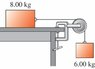# The Work - Energy Theorem in a pulley?

erik-the-red
Consider the system shown in the figure. The rope and pulley have negligible mass, and the pulley is frictionless. The coefficient of kinetic friction between the 8.00-kg block and the tabletop is $$\mu_k=0.250.$$ The blocks are released from rest.

Use energy methods to calculate the speed of the 6.00-kg block after it has descended 1.50 m.

$$\Delta * K$$ = $$(1/2)(m)(v_f^2 - v_i^2)$$

But, it was at rest, so $$(1/2)(m)(v_f^2)$$ is what matters.

What do I do next?

#### Attachments

•Imajj.jpg
7 KB · Views: 589